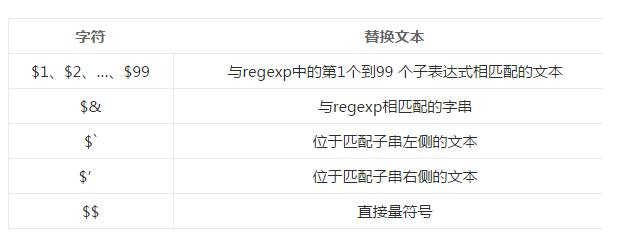# js正则表达式学习和总结(必看篇)

更新时间：2016年11月22日 08:25:47   投稿：jingxian我要评论

### （推荐）JS正则知识点专题：//www.jb51.net/article/139831.htm

var reg = /pattern/attributes

var reg = new RegExp(pattern, attributes);

1.采用直接量语法新建的正则表达式对象在代码编译时就会生成，是平常开发中常用的方式；
2.采用构造函数生成的正则对象要在代码运行时生成。

ignoreCase 返回布尔值，表示RegExp 对象是否具有标志 i
global 返回布尔值，表示RegExp对象是否具有标志g
multiline 返回布尔值，表示RegExp 对象是否具有标志 m。
lastIndex 一个整数，标识开始下一次匹配的字符位置
source 返回正则表达式的源文本（不包括反斜杠）

i 执行对大小写不敏感的匹配

g 执行全局匹配（查找所有匹配而非在找到第一个匹配后停止）。
m 执行多行匹配

1.验证

2.搜索替换

[…] 查找方括号之间的任何字符
[^…] 查找任何不在方括号之间的字符
[a-z] 查找任何从小写 a 到小写 z 的字符
[A-Z] 查找任何从大写 A 到大写 Z 的字符
[A-z] 查找任何从大写 A 到小写 z 的字符
. 查找单个字符，除了换行和行结束符
\w 查找单词字符，等价于[a-zA-Z0-9]
\W 查找非单词字符，等价于[^a-zA-Z0-9]
\s 查找空白字符
\S 查找非空白字符
\d 查找数字，等价于[0-9]
\D 查找非数字字符，等价于[^0-9]
\b 匹配单词边界
\r 查找回车符
\t 查找制表符
\0 查找 NULL 字符
\n 查找换行符

{n,m} 匹配前一项至少n次，但不能超过m次
{n,} 匹配前一项n次或更多次
{n} 匹配前一项n次
n？ 匹配前一项0次或者1次，也就是说前一项是可选的，等价于{0，1}
n+ 匹配前一项1次或多次，等价于{1，}
n* 匹配前一项0次或多次，等价于{0，}
n\$ 匹配任何结尾为 n 的字符串
^n 匹配任何开头为 n 的字符串
?=n 匹配任何其后紧接指定字符串 n 的字符串
?!n 匹配任何其后没有紧接指定字符串 n 的字符串

^[1-9]\d*\$　 　 匹配正整数
^-[1-9]\d*\$ 　 匹配负整数
^-?[0-9]\d*\$　　 匹配整数
^[1-9]\d*|0\$　 匹配非负整数（正整数 + 0）
^-[1-9]\d*|0\$　　 匹配非正整数（负整数 + 0）
^[1-9]\d*.\d*|0.\d*[1-9]\d*\$　　匹配正浮点数
^-([1-9]\d*.\d*|0.\d*[1-9]\d*)\$　匹配负浮点数
^-?([1-9]\d*.\d*|0.\d*[1-9]\d*|0?.0+|0)\$　 匹配浮点数
^[1-9]\d*.\d*|0.\d*[1-9]\d*|0?.0+|0\$　　 匹配非负浮点数（正浮点数 + 0）
^(-([1-9]\d*.\d*|0.\d*[1-9]\d*))|0?.0+|0\$　　匹配非正浮点数（负浮点数 + 0）

^[A-Za-z]+\$　　匹配由26个英文字母组成的字符串
^[A-Z]+\$　　匹配由26个英文字母的大写组成的字符串
^[a-z]+\$　　匹配由26个英文字母的小写组成的字符串
^[A-Za-z0-9]+\$　　匹配由数字和26个英文字母组成的字符串
^\w+\$　　匹配由数字、26个英文字母或者下划线组成的字符串

test方法

Demo1:

```<!DOCTYPE html>
<html>
<meta charset="utf-8">
<meta http-equiv="X-UA-Compatible" content="IE=edge">
<title>test方法</title>
<body>
<script type="text/javascript">
var reg = /abc/g;
var str = "123abc456abc";
console.log(reg.lastIndex);//0
console.log(reg.test(str));//true
console.log(reg.lastIndex);//6
console.log(reg.test(str));//true
console.log(reg.lastIndex);//12
console.log(reg.test(str));//false
</script>
</body>
</html>```

Demo2:

```<!DOCTYPE html>
<html>
<meta charset="utf-8">
<meta http-equiv="X-UA-Compatible" content="IE=edge">
<title>test方法</title>
<body>
<script type="text/javascript">
console.log(new RegExp('').test('abc'));//true
console.log(/''/.test('abc'));//false
console.log(/''/.test("''"));//true
</script>
</body>
</html>```

exec方法

exec() 方法用于检索字符串中的正则表达式的匹配。

Demo1:

```<!DOCTYPE html>
<html>
<meta charset="utf-8">
<meta http-equiv="X-UA-Compatible" content="IE=edge">
<title>exec方法</title>
<body>
<script type="text/javascript">
var str = "xyz";
var reg1 = /x/;
var reg2 = /a/;
var res1 = reg1.exec(str);
var res2 = reg2.exec(str);
console.log(res1);//["x", index: 0, input: "xyz"]
console.log(res2);//null
</script>
</body>
</html>```

Demo2:

```<!DOCTYPE html>
<html>
<meta charset="utf-8">
<meta http-equiv="X-UA-Compatible" content="IE=edge">
<title>exec方法2</title>
<body>
<script type="text/javascript">
var str = 'abcdabc';
var reg = /(a)b(c)/;
var res = reg.exec(str);
console.log(res);//["abc", "a", "c", index: 0, input: "abcdabc"]
</script>
</body>
</html>```

input 整个原待匹配的字符串
index 整个模式匹配成功的开始位置

search方法

search() 方法用于检索字符串中指定的子字符串，或检索与正则表达式相匹配的子字符串。

search() 方法不执行全局匹配，它将忽略标志 g。它同时忽略 regexp 的 lastIndex 属性，并且总是从字符串的开始进行检索，这意味着它总是返回 stringObject 的第一个匹配的位置。

Demo:

```<!DOCTYPE html>
<html>
<meta charset="utf-8">
<meta http-equiv="X-UA-Compatible" content="IE=edge">
<title>search方法</title>
<body>
<script type="text/javascript">
var str = "abcdcef";
console.log(str.search(/c/g));//2
</script>
</body>
</html>```

match方法

match() 方法可在字符串内检索指定的值，或找到一个或多个正则表达式的匹配。该方法类似 indexOf() 和 lastIndexOf()，但是它返回指定的值，而不是字符串的位置。

Demo:

```<!DOCTYPE html>
<html>
<meta charset="utf-8">
<meta http-equiv="X-UA-Compatible" content="IE=edge">
<title>match方法</title>
<body>
<script type="text/javascript">
var str = "abcd";
var reg1 = /a/;
var reg2 = /x/;
console.log(str.match(reg1));//["a", index: 0, input: "abcd"]
console.log(str.match(reg2));//null

var str = "abcdabc";
var reg = /a/g;
console.log(str.match(reg));//["a", "a"]
console.log(reg.exec(str));//["a", index: 0, input: "abcdabc"]
</script>
</body>
</html>```

replace方法

replace() 方法用于在字符串中用一些字符替换另一些字符，或替换一个与正则表达式匹配的子串。

Demo:

```<!DOCTYPE html>
<html>
<meta charset="utf-8">
<meta http-equiv="X-UA-Compatible" content="IE=edge">
<title>replace方法</title>
<body>
<script type="text/javascript">
var str = "xxx";
console.log(str.replace('x','y'));//yxx
console.log(str.replace(/x/,'y'));//yxx
console.log(str.replace(/x/g,'y'));//yyy
</script>
</body>
</html>```

replace方法中特殊字符替换Demo:

```<!DOCTYPE html>
<html>
<meta charset="utf-8">
<meta http-equiv="X-UA-Compatible" content="IE=edge">
<title>replace中的特殊字符替换</title>
<body>
<script type="text/javascript">
//用子表达式替换：\$1和\$2
//正则表达式中()就是一个子表达式，\$1对应是第一个表达式的内容，即java，\$2为script
var str = "javascript";
console.log(str.replace(/(java)(script)/,'\$2\$1')); //输出：scriptjava
//\$& 为正则表达式匹配的字符串
//正则表达式通过直接量java来匹配，匹配结果为java，则 \$&的值为java，然后用字符串\$&-来替换匹配的字符串
var str1 = "javascript";
console.log(str1.replace(/java/,'\$&-')); //输出：java-script

var str2 = "javascript";
// \$`为匹配子串ava的左侧文本，则为j
console.log(str2.replace(/ava/,"\$`")); //输出：jjscript
// \$'为匹配子串ava的右侧文本，则为script
console.log(str2.replace(/ava/,"\$'")); //输出：jscriptscript
// \$\$为直接量符号，即插入一个\$符号
console.log(str2.replace(/ava/,"\$\$"));//输出：j\$script
</script>
</body>
</html>```

replace的参数replacement是函数

match 为匹配整个字符串，即：xyz45678%\$&^
a1 为第一个子表达式，([^\d]*)，匹配0个或多个非数字的字符，即:xyz
a2 为第二个子表达式,(\d*)，匹配0个或多个的数字，即：45678
a3 为第三个子表达式，([^\w]*)，匹配0个或匹配任何非单词字符。等价于 [^A-Za-z0-9_]，即 %\$&^
index为模式匹配出现的位置，从第一个字符已经匹配成功，则位置为0
string为字符串本身，即 xyz45678%\$&^

Demo:

```<!DOCTYPE html>
<html>
<meta charset="utf-8">
<meta http-equiv="X-UA-Compatible" content="IE=edge">
<title>replace的参数replacement是函数</title>
<body>
<script type="text/javascript">
function replacer(match, a1, a2, a3, index, string) {
return [a1, a2, a3].join(' ~ ');
}
var str = 'xyz45678%\$&^';
var reg = /([^\d]*)(\d*)([^\w]*)/
var res = str.replace(reg, replacer);
console.log(res);//xyz ~ 45678 ~ %\$&^
</script>
</body>
</html>```

split方法

split(‘字符串的分割正则','返回数组的最大成员数')；返回分割后各部分组成的数组
Demo:

```<!DOCTYPE html>
<html>
<meta charset="utf-8">
<meta http-equiv="X-UA-Compatible" content="IE=edge">
<title>split方法</title>
<body>
<script type="text/javascript">
var str = 'a,b , c,d';
var res = str.split(",");//以逗号来分割字符串
console.log(res);//["a", "b ", " c", "d"]

var str1 = 'a,b , c,,d';
var res1 = str1.split(/,*/);//以0或多个逗号来分割字符串
console.log(res1);//["a", "b", " ", " ", "c", "d"]

var str2 = 'a, b,c, d';
var res2 = str2.split(/, */);//以0或对个逗号空格来分割字符串
console.log(res2);//["a", "b", "c", "d"]

var str3 = 'a, b,c, d';
var res3 = str3.split(/, */,2);//以0或对个逗号空格来分割字符串，同时限制返回数组中最多有两项
console.log(res3);//["a", "b"]
</script>
</body>
</html>```

Demo2:

```<!DOCTYPE html>
<html>
<meta charset="utf-8">
<meta http-equiv="X-UA-Compatible" content="IE=edge">
<title>split方法2</title>
<body>
<script type="text/javascript">
var str = "x@@xx@xx@@";
var res = str.split(/x*/);//以0或者对个x为分隔符
console.log(res);//["", "@", "@", "@", "@", "@"]

var res1 = str.split(/(x*)/);//如果加上括号则括号匹配的部分也就是分割规则也会作为数组成员返回
console.log(res1);//["", "x", "@", "", "@", "xx", "@", "xx", "@", "", "@"]
</script>
</body>
</html>```

1.字符串中出现次数最多的字符

var re = /(\w)\1+/g;
(\w)外面的圆括号表示分组,\1表示重复第一分组中的内容 ，\1+表示 \w匹配到的字符重复n次，后面的g表示执行全部替换

str.replace的第二个参数是个函数,参数a表示整个匹配到的字符串，b表示第一捕获分组也就是出现重复的单个字符 ，将a.length 与已经记录 过的最多重复num比较，如果a.length 更大，就将它赋值给num,用value记录重复字符 b，这个函数返回的是替换文本，但这里没有返回值，也就是说替换 为空，每次替换这个函数都被执行

```<!DOCTYPE html>
<html>
<meta charset="utf-8">
<meta http-equiv="X-UA-Compatible" content="IE=edge">
<title>字符串中出现次数最多的字符</title>
<body>
<script type="text/javascript">
var str = 'mmmmmmmmaaammmmmmmmmbbbbsccc';
function most(str) {
var arr = str.split('');
str = arr.sort().join('');//将字符串按单个字符分割，然后排序组合，经过这一步，相同的字符就会排列到一起
var reg = /(\w)\1+/g;
var num = 0;
var value = '';
str.replace(reg, function (a,b) {
// console.log(a);
if (num<a.length) {
num = a.length;
value = b;
}
});
return '出现次数最多的字符是' + value + '出现了' + num + '次';
}
console.log(most(str));
</script>
</body>
</html>```

2.从url中提取子域名

```<!DOCTYPE html>
<html>
<meta charset="utf-8">
<meta http-equiv="X-UA-Compatible" content="IE=edge">
<title>从url中提取子域名</title>
<body>
<script type="text/javascript">
var url = "http://www.abc.com";
var reg = /[^.]+/;//匹配除了.之外的其他字符
var res = reg.exec(url).substr(7);
console.log(reg.exec(url));//["http://www", index: 0, input: "http://www.abc.com"]
console.log(res);//www
</script>
</body>
</html>```

3.给字符串加千分符

```<!DOCTYPE html>
<html>
<meta charset="utf-8">
<meta http-equiv="X-UA-Compatible" content="IE=edge">
<title>给字符串加千分符</title>
<body>
<script type="text/javascript">
var str = "12345678912312";
function fn(str){
var reg = /(?=(?!b)(\d{3})+\$)/g;
return str.replace(reg,',');
}
var res = fn(str);
console.log(res);//12,345,678,912,312
</script>
</body>
</html>```

/^([a-zA-Z_0-9-])+@([a-zA-Z_0-9-])+(.[a-zA-Z_0-9-])+\$/

/^\d*.?\d{0,2}\$/

\w是 匹配英文，数字，下划线